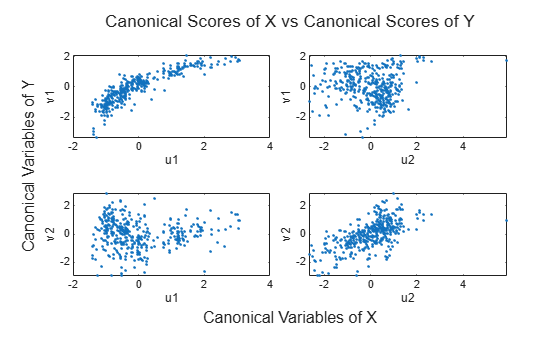Documentation

This is machine translation

Mouseover text to see original. Click the button below to return to the English version of the page.

canoncorr

Canonical correlation

Syntax

```[A,B] = canoncorr(X,Y) [A,B,r] = canoncorr(X,Y) [A,B,r,U,V] = canoncorr(X,Y) [A,B,r,U,V,stats] = canoncorr(X,Y) ```

Description

`[A,B] = canoncorr(X,Y)` computes the sample canonical coefficients for the `n`-by-`d1` and `n`-by-`d2` data matrices `X` and `Y`. `X` and `Y` must have the same number of observations (rows) but can have different numbers of variables (columns). `A` and `B` are `d1`-by-`d` and `d2`-by-`d` matrices, where `d = min(rank(X),rank(Y))`. The `j`th columns of `A` and `B` contain the canonical coefficients, i.e., the linear combination of variables making up the `j`th canonical variable for `X` and `Y`, respectively. Columns of `A` and `B` are scaled to make the covariance matrices of the canonical variables the identity matrix (see `U` and `V` below). If `X` or `Y` is less than full rank, `canoncorr` gives a warning and returns zeros in the rows of `A` or `B` corresponding to dependent columns of `X` or `Y`.

`[A,B,r] = canoncorr(X,Y)` also returns a 1-by-`d` vector containing the sample canonical correlations. The `j`th element of `r` is the correlation between the jth columns of `U` and `V` (see below).

`[A,B,r,U,V] = canoncorr(X,Y)` also returns the canonical variables, scores. `U` and `V` are `n`-by-`d` matrices computed as

```U = (X-repmat(mean(X),N,1))*A V = (Y-repmat(mean(Y),N,1))*B```

`[A,B,r,U,V,stats] = canoncorr(X,Y) ` also returns a structure `stats` containing information relating to the sequence of `d` null hypotheses ${H}_{0}^{\left(k\right)}$, that the (`k+1`)st through `d`th correlations are all zero, for ```k = 0:(d-1)```. `stats` contains seven fields, each a `1`-by-`d` vector with elements corresponding to the values of `k`, as described in the following table:

FieldDescription
`Wilks`

Wilks' lambda (likelihood ratio) statistic

`df1`

Degrees of freedom for the chi-squared statistic, and the numerator degrees of freedom for the F statistic

`df2`

Denominator degrees of freedom for the F statistic

`F`

Rao's approximate F statistic for ${H}_{0}^{\left(k\right)}$

`pF`

Right-tail significance level for `F`

`chisq`

Bartlett's approximate chi-squared statistic for ${H}_{0}^{\left(k\right)}$ with Lawley's modification

`pChisq`

Right-tail significance level for `chisq`

`stats` has two other fields (`dfe` and `p`) which are equal to `df1` and `pChisq`, respectively, and exist for historical reasons.

Examples

collapse all

```load carbig; X = [Displacement Horsepower Weight Acceleration MPG]; nans = sum(isnan(X),2) > 0;```

Compute the sample canonical correlation.

`[A,B,r,U,V] = canoncorr(X(~nans,1:3),X(~nans,4:5));`

Plot the canonical variables scores.

```plot(U(:,1),V(:,1),'.') xlabel('0.0025*Disp+0.020*HP-0.000025*Wgt') ylabel('-0.17*Accel-0.092*MPG')```References

 Krzanowski, W. J. Principles of Multivariate Analysis: A User's Perspective. New York: Oxford University Press, 1988.

 Seber, G. A. F. Multivariate Observations. Hoboken, NJ: John Wiley & Sons, Inc., 1984.